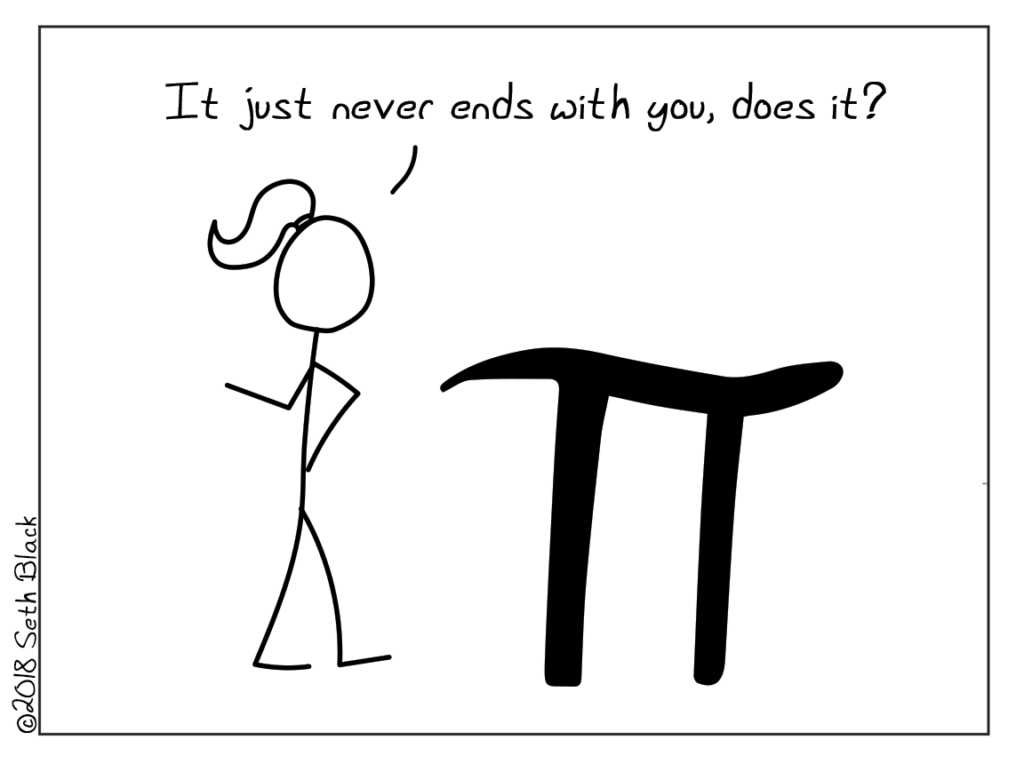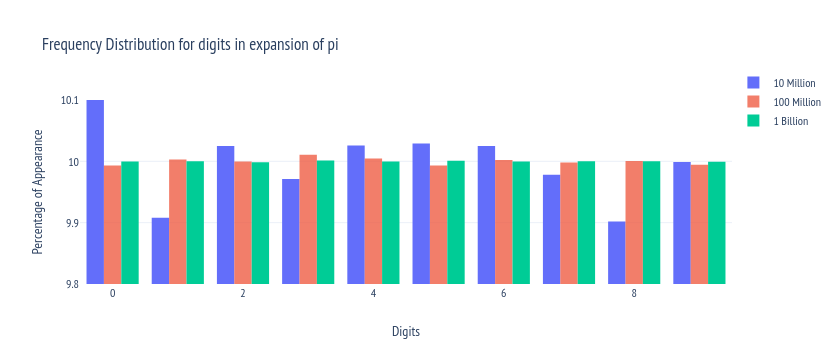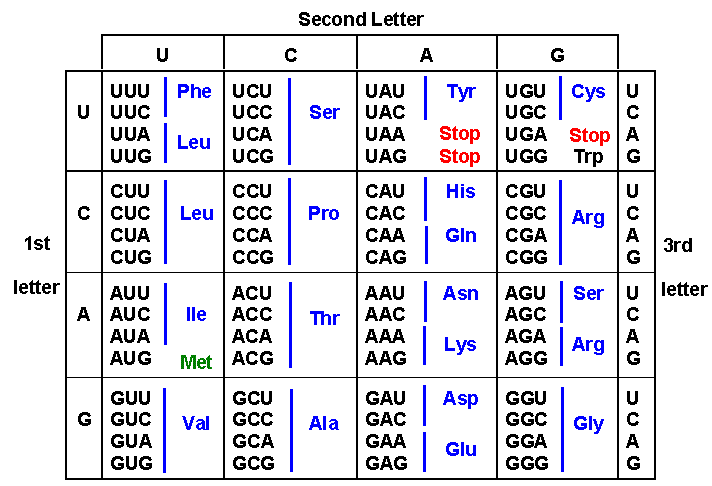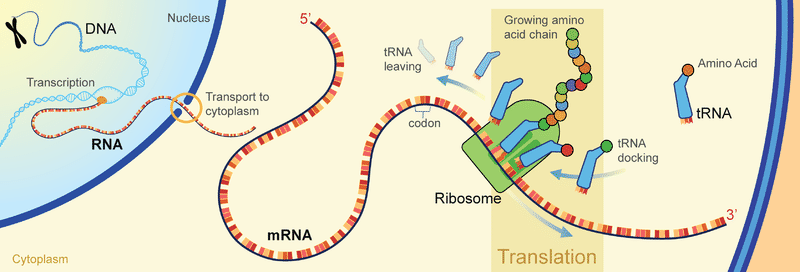Continuing my tradition of writing a blog post every pi day (14th March 3/14 , is celebrated as pi day every year). Today we will be discussing about the decimal expansion of pi and a class of numbers that are known as Normal Numbers. We will also be dealing with some minor biology and information theory. So sit back and enjoy.

I am pretty sure you have heard of it before. It is one of the most famous number in the world. π is a ratio that we come across if we study mathematics even at an elementary Level. You must already know that it has an unending infinite decimal expansion (Explored in previous post on how to calculate pi) . In this post we will talk about the beauty of pi in detail (See last year's pi day post about surprising places pi appears in), discuss what are normal numbers and show (not prove) that π most probably is a normal number.

I have used python for analysing more than 100 Million Digits of Pi to show the Randomness and behaviour of pi and analysing the "Normalness" of the Number.

We will also talk about why I believe , you, me and everything in the universe can exist in the unending digits of π.

## ${\pi}$

### Life of Pi

Defined as the ratio of the circumference of a circle to its diameter, pi, or in symbol form, π, seems a simple enough concept. Although the number and it's value was calculated way back in 2000 BCE by Early babilonians and Indian philosophers (Indian sources by about 150 BC treat $\pi$ as $\sqrt{10}$ ≈ 3.1622. wiki).The extent of the decimal expansion for the irrational number has gone up exponentially as more and more advancements have been made to machines. Many computer scientists took part in a race to calculate maximum digits in the expansion of pi. Recently the record is held by Timothy Mullican (USA) who calculated 50,000,000,000,000 digits using old server equipment and a software called y cruncher.(Most accurate value of $\pi$ Guinness World Records (link)

Here are first 100 digit of pi in base 10:

3.14159265358979323846264338327950288419716939937510582097 4944592307816406286208998628034825342117067

Above you can see I have colored few numbers separately. It is not just for aesthetic purposes(Although the colors do look pretty). I have separately colored digits 1,2,3,and 4 so that you can count the number of times they appear. 1 occurs 8 times, 2 appears 12 times while 3 gets repeated 10 times, and 4 appears 10 times again. If we take mean frequency of each digit appearing, we find it close to 10 times for 100 digits, giving a frequency of 0.1. Infact the table below provides you the value for all 10 digits: And you can see the distribution is more or less flat. Meaning If i were to pick up a digit randomly from the 100 digits written above, chances of the digit being 0 is equally likely than it being any other digit. This is known as uniformly distributed numbers.

+--------+----------------------------+------------+
| Number | Number of times it appears | Percentage |
+--------+----------------------------+------------+
|  ones  |             8              |    8.0     |
|  twos  |             12             |    12.0    |
| threes |             11             |    11.0    |
| fours  |             10             |    10.0    |
| fives  |             8              |    8.0     |
| sixes  |             9              |    9.0     |
| sevens |             8              |    8.0     |
| eights |             12             |    12.0    |
| nines  |             14             |    14.0    |
| zeroes |             8              |    8.0     |
+--------+----------------------------+------------+
Number of Decimal Places Considered: 100

Most of you can already see where this is leading to. Let us now introduce the concept of normal numbers and see how pi fits in there.

### Normal Numbers, Is pi normal?

For a non mathematician, normality of a number or abnormality of a number may sound absurd. It did to me when i first read about it 5 years ago. It was when i started writing this post and started my spiraling obsession with this number. Since then, the world record for expansion of pi has been broken multiple times. And my thirst of curiosity has not yet been quenched. Let us take a step back from the decimal expansion of pi and discuss what Normal Number actually is.

Wolfram Mathworld defines normal number as :

A number is said to be simply normal to base $b$ if its base- $b$ expansion has each digit appearing with average frequency tending to $b^{-1}$ (Wolfram MathWorld Normal Numbers (link)

In simple words, if the number in in base 10 (digits 0 to 9), each digit will appear with frequency $\frac{1}{10}$ or 0.1. Infact the frequency of finding a number that is k digits long is given by $b^{-k}$. So frequency of occurrence of objects like '12', '01','99' ,etc. has a frequency of $\frac{1}{10^2} = 0.01$ in decimal (base 10) system. And numbers like '123','078','569',etc has a frequency of $\frac{1} {10^3} =0.001$.

It is an unsolved problem in Mathematics to prove that irrational numbers like $\pi$,$\sqrt{2}$, e or $\sqrt{s}$ for any s is a normal number or not. But we can still verify with whatever data that we have got if it is a normal number or not.

A few years ago I started looking at ways to calculate python to large number of digits. Unfortunately, soon i landed on an issue. And the issue (as always) turned out to be money. Or lack thereof. Perhaps this shouldn't be a surprise, that to calculate pi-to say 100 million or a billion digits- it requires heavy expensive hardware. A few tens of thousand can be calculated in any usual machine. (More advanced laptops can even calculate million digits of pi using clever techniques).The internet as usual came to the rescue. You can download the expansion of pi online via multiple resources. If you really want to go crazy there is a 22 trillion digits datasets available online (He also provides smaller datasets at his blog). I used this MIT's database(see the reference) (MIT's database https://stuff.mit.edu/afs/sipb/contrib/pi/ with billion digits of pi) for billion digits of pi.

I wrote a very simple code to count number of digits in the data file.All Codes are in the GitHub repository in the references below (GitHub Repository). (The repository has similar analysis code for $\sqrt{2}$ and e to check normality of the numbers)

Following table shows the result of code that processes a billion digits of pi. It took my not so decent laptop around 727 seconds (more than 12 minutes) to process all billion digits of pi.

 Digit 1 2 3 4 5 6 7 8 9 0 # occurred 99997334 100002410 99986911 100011958 99998885 100010387 99996061 100001839 100000273 99993942
Distribution of digits in billion digit expansion of pi

The following chart provides results for 10 million, 100 million and 1 Billion digits of Pi. As we increase the number of digits, the percentage gets flattened out. We can do same analysis for finding two digit strings (like '01','12',etc.) and we will find the same uniform distribution now with probability staying around 1Testing Normality of pi

Let us take it a step ahead. We know that from a uniformly distributed set, if we pick up a number it is equally likely to be any number. Can we say then that it is a completely random distribution. Yes, infact people have shown that digits of pi (with scrambling) can be used as better random number generators than what we already use in our machines. (Using π digits to Generate Random Numbers: A Visual and Statistical Analysis (arxiv link).

As we have shown in a normal number any set of k numbers follows the frequency 1/$b^{k}$. By definition, We can find any arbitrary set of number in the decimal expansion of pi (assuming we have appropriate number of digits). To be clear, if frequency of occurrence is 1/x, then we need more than x digits to get the set at least once in the expansion. (The mathematics of finding set of numbers in expansion goes beyond this and deals with binomial distribution) Take some time and let that sink in. It really blew my mind when I first realized it. For example, we can find first few digits of pi in the expansion of pi itself.

I did exactly this in another program, this program searches for a string of number (14159265 to be exact from $\pi ~3.14159265$ )(pi_normal1.py in GitHub Repository). As expected we get the number '1' ($\frac{1}{10^1} \times 10^7$) almost a million times. String like '1415' is found ~1000 times as expected using $\frac{1}{10^4} \times 10^7 = 10^3=1000$. A 8 digit long string should not exist in the expansion with $10^7$ digits, but here one such string exists. Can you think of reason why? It is quite an obvious one. (Hint: Same reason exists for why a 7 digit long string is occurring twice and not once.)

(base) admin@laptop:~/Desktop/pi/Python\$ python pi_normal1.py
No. of Digits Considered:  10000000
+----------+--------------------------+
| String   | No. of times it appears  |
+----------+--------------------------+
|    1     |          999333          |
|    14    |          100232          |
|   141    |          10158           |
|   1415   |           1001           |
|  14159   |            98            |
|  141592  |            9             |
| 1415926  |            2             |
| 14159265 |            1             |
+----------+--------------------------+
1415926 found at position : 1457054

--- 18.550782918930054 seconds ---

18.550782918930054 seconds –- 

## Library of Babel

Jorge Luis Borges in 1941 wrote a short story called Library of Babel (Original text translated in english ), the following quote is extracted from the story itself.

Everything: the minutely detailed history of the future, the archangels' autobiographies, the faithful catalogues of the Library, thousands and thousands of false catalogues, the demonstration of the fallacy of those catalogues, the demonstration of the fallacy of the true catalogue, the Gnostic gospel of Basilides, the commentary on that gospel, the commentary on the commentary on that gospel, the true story of your death, the translation of every book in all languages, the interpolations of every book in all books.

The Library of Babel,by Jorge Luis Borges(1941)

In the library of Babel, Borges imagines a library made up of hexagonal rooms, each hexagonal room having walls covered with shelves. Each book shelf containing volumes and volumes of books. Each book containing 410 pages completely filled with text. The library of Babel has all possible combinations of the 25 characters that are possible in the language(the story is being told in), including comma, fullstop and space. In the library any string of letter, any collection of word that was ever written or will ever be written hides in seemingly infinite books.

The philosophical themes underlying the library is immense. What is the purpose of writer as a creator, if what he writes has already been thought of. Is any thought original? From the questions of free will, to the idea of reality, library of babel is a gold mine for human thought. You can explore the library of babel using the link in the reference and have your mind blown by the absurdity of human thought, (Libraryofbabel.info created by Jonathan Basile Emory Comp Lit Ph.D. Candidate).

But you might be wondering why we are talking about it in a post about pi?

I would like to call pi, as a kind of library of babel itself. Only the set of characters have changed. Assuming we could transform text into base 10 decimal number, and ignoring comma, spaces and full stop, we can potentially find every possible string ever inside the library of Babel.

Library of Babel has also been compared with the human genome and protein sequence. Much like the library of Babel, the protein (peptide) sequence in living thing forms seemingly arbitrary chain.

### Human Genome and Pi

Let us take some time and discuss some biology. Living beings (may it be humans, bacteria or plants) are made up of cells. Each cell has a nucleus in the center and inside the nucleus lies the genetic material of the cell. It is made up of heavily condensed long chain polymers of Nucleic Acids. The polymer are bound together in the famous double helical structure, which is known as DNA.

There are four kinds of Nucleotide bases(which makes up the polymer-DNA) Adenine (A), Thymine (T), Guanine (G) and Cytosine (C), the other strand of the DNA has complimentary bases, A joins with T and G joins with C.

This string of bases, A,T,G and C forms the genetic information of a living being. It provides all information that is needed for the cell to function. Let us consider an example of protein formation to understand it. Each protein is coded by a sequence of Genetic bases. The sequence is known as a codon. A codon consists of three bases. There are total (4*4*4) 64 codons which code for 20 known Amino Acids (Protein building blocks).These codon correspond with RNA so you can replace the base U with T to read the codons on the DNA

Let us say the body is in need of insulin, the cell sends signal to the DNA and a complicated yet enthralling process of Translation starts. In the process of Translation, DNA gets unwound and turns into a single stranded mRNA (messenger RNA-Ribonucleic Acid), the RNA comes out of the nucleus and Ribosome(a machine that moves on the strand) reads the RNA and produces chain of Amino Acids that correspond to the code on the RNA. Actual process is a bit more complicated but beyond the scope of the article.Translation mechanism

Now let's get back to our favourite number $\pi$ and its expansion. Let us consider an example of a particular gene in our body. Human beings require Insulin to function. Insulin generation is controlled by a gene (with gene_id=3630 from NCBI database). The gene for insulin consists of 465 Bases and produces peptide of length 110. Let us see how we can convert from the genetic code to the digits of pi.

We can let each base to be a digit in base 4 numeric system. Say A=0, T=1, G=2 and C=3. Now each genetic code can be converted into a string of number in base 4. We can then convert base 4 to base 10 using simple method. A better way to handle it would be to convert the codons into decimal directly using basae 4 to base 10 conversion. In the following code block we can see each of the possible codon(even those that do not code for proteins) and its corresponding numeric value in decimal system.

change={
'genome':['aaa','aat','aag','aac',
'ata','att','atg','atc',
'aga','agt','agg','agc',
'aca','act','acg','acc',
'taa','tat','tag','tac',
'tta','ttt','ttg','ttc',
'tga','tgt','tgg','tgc',
'tca','tct','tcg','tcc',
'gaa','gat','gag','gac',
'gta','gtt','gtg','gtc',
'gga','ggt','ggg','ggc',
'gca','gct','gcg','gcc',
'caa','cat','cag','cac',
'cta','ctt','ctg','ctc',
'cga','cgt','cgg','cgc',
'cca','cct','ccg','ccc'],
'converted':
[ 0,  1,  2,  3,  4,  5,  6,  7,  8,  9, 10, 11, 12, 13, 14, 15, 16,
17, 18, 19, 20, 21, 22, 23, 24, 25, 26, 27, 28, 29, 30, 31, 32, 33,
34, 35, 36, 37, 38, 39, 40, 41, 42, 43, 44, 45, 46, 47, 48, 49, 50,
51, 52, 53, 54, 55, 56, 57, 58, 59, 60, 61, 62, 63],
}`

Let us now consider say the insulin gene. From base #441 to base #450, the code starts with the start codon and ends with the stop codon.

Bases: atggaataa (This codes for Glutamic Acid) base10_decimal conversion: 63216 (This string occurs 1982 times in the first 200M digits of Pi)( Search your own string in pi)

If we add two more successive codons we get 632161161, This string occurs 1 times in the first 200M digits of Pi.

Since we know, and have analytic proof that the decimal expansion pi is non repeating and non recurring (irrational number). By definition we can keep increasing the size of gene and keep calculating the digits of pi and we will find the genetic code inside the expansion of pi. The complete Insulin gene would translate to a 296 digit long decimal number and to find an instance of it we would need to calculate $10^{296}$ digits of pi.

I know, I know it sounds absurd. But it is hypothetically correct.

Many might consider it a stretch, but so far people have explored trillions of digits of pi and the normality is here to stay.(Digit Statistics of the First $\pi^e$ Trillion Decimal Digits of π :arxiv link). And in future if some brilliant mathematician can prove the normality of pi analytically, we can safely say any string of digits would lie in the expansion of pi. So assuming instead of having the words and letters in the volumes in the library, we could make a library with shelves lined up the wall made of decimal expansion of $\pi$. While library of babel is a finite library (that leaves me as a writer breathless- there is a limit to human thought) the pi library would be infinite. And among those, a wondering librarian might find a book shelf that would consist of you. Your essence of being, every base pair from your DNA would be written in that bookshelf. And so would be mine. An amazing thought to think about and to end with.

title: "Love Letters to pi |Do you exist in pi? Normality of $\pi$" date: "2021-03-14" categories:

• "mathematics"

• "physics"

tags:

• "mathematics"

• "philosophy"

• "physics"

• "pi-day"

coverImage: "Explore-the-Mysterious-Number-Pi-π-Cover-Picture-820x461-1.jpg"